Next: B-Production Facilities Up: CP Violation and Future Previous: CP Violation and Future

# CP Violation in the B-system

Quark mixing in the standard model is described by the Cabibbo-Kobayashi-Maskawa (CKM) matrix , eqn(1). Conventionally the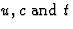quarks are unmixed and the mixing is described by the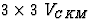matrix operating on the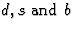quarks. The matrix elements of VCKM can, in principle, be determined by measuring the charged current coupling to the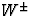bosons.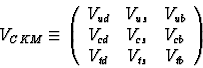(1)

The CKM matrix is unitary ie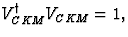which leads to 9 unitarity conditions expressed in terms of the matrix elements. There are several (approximate) parameterisations of the CKM matrix, one of the more popular approaches is that of Wolfenstein , eqn(2), where the matrix elements are expressed in terms of powers of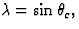whereis the Cabibbo angle. As can be seen from this parameterisation, the 9 complex elements of the matrix can be expressed in terms of 4 independent variables; three real parameters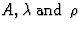and an imaginary part of a complex number,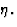The 18 parameters of the CKM matrix can be reduced to 4 because of the unitarity constraints and the arbitrary nature of the relative quark phases . It is the complex phase in the VCKM that leads to CP violation in the standard model.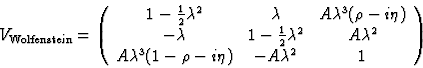(2)

The unitarity condition

VudVub* + VcdVcb* + VtdVtb* = 0

is of particular interest since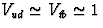and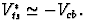This allows us to depict this condition as a triangle in the complex plane, as shown in fig 1. The angles of the triangle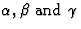are related to the phase and can be measured in CP violating B-decays.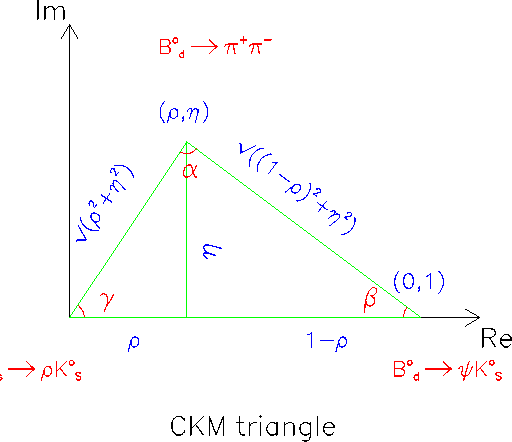The non-closure of this triangle ie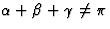would suggest that our understanding of CP violation within the Standard Model was incomplete. Physics beyond the Standard Model can be further investigated, for example, by measuring CP asymmetries in several B decays that depend on the same unitarity angle or studying decays where zero asymmetries are expected in the Standard Model.

CP violation in the B system should be observable through the phenomenon of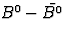mixing, see for example . Thismixing is dominated by box-diagrams with virtual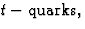fig 2.The following decays

are into a CP eigenstate. If this is coupled with only a single diagram contributing to the decay, CP asymmetries can be constructed which are directly related to the angles of the unitarity triangle. For example, these conditions occur for the decay mode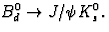Here the number of B0d which decay at time t (where t is expressed in units of lifetime) is proportional to(3)
and the number ofis proportional to(4)
where the mixing parameter,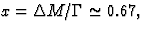is the ratio of the mass difference of the eigenstates to their decay rate. The CP asymmetry can then be defined asBy integrating eqns. (4) and (5) over time a similar asymmetry can be constructed which is proportional to. (Although for coherent B production ie thepair is produced in a definite CP state, this time integrated asymmetry is zero.) In addition this channel is experimentally very promising because of the dilepton decay of the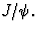Unfortunately additional decay diagrams contribute to the other channels listed in eqn(3) so there is no longer a complete cancellation of the hadronic matrix elements in the CP asymmetry. The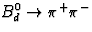channel, which is dependent on the angleis predicted to have large hadronic corrections from `penguin' diagrams, fig. 3. The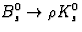(dependent on the angle) also has additional hadronic contributions but, in addition, suffers from a very low branching fraction. Fortunately there are, of course, many other channels which can be used to measure CP violation, eg see ref. .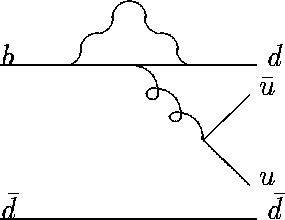Next: B-Production Facilities Up: CP Violation and Future Previous: CP Violation and Future

latex2html conversion by www person on 1997-10-22